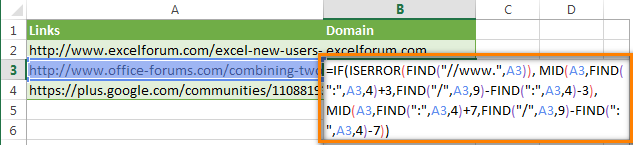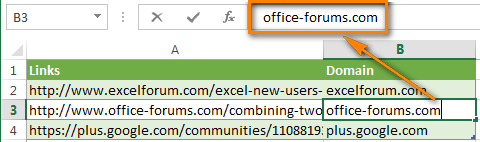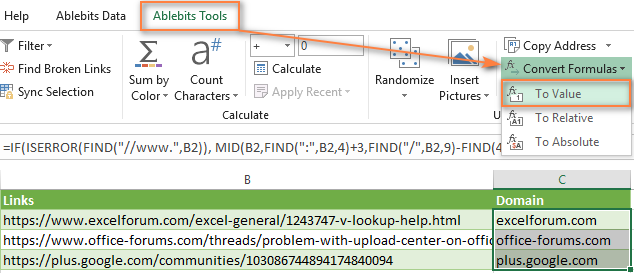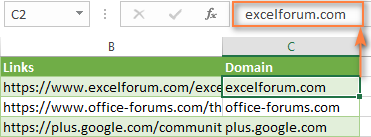# How to replace formulas with their values in Excel

Here are good tips to save your time - 2 fastest ways to replace formulas in Excel cells with their values. Both hints work for Excel 365 - 2013.

You may have different reasons for converting formulas to values:

• To be able to quickly insert values to other workbooks or sheets without wasting time on copy/paste special.
• To keep your original formulas unknown when you send a workbook to another person (for example, your retail markup to the wholesale price).
• To prevent the result from modifying when the numbers in the linking cells change.
• Save the result of the rand() formula.
• If you have a lot of complex formulas in your workbook which make recalculating really slow. And you cannot switch the "Workbook calculation" option to the manual mode.

## Converting formulas to values using Excel shortcuts

Suppose, you have the formula to extract domain names from URLs.

You need to replace its results with values.Just follow the simple steps below:

1. Select all the cells with formulas that you want to convert.
2. Press Ctrl + C or Ctrl + Ins to copy formulas and their results to clipboard.
3. Press Shift + F10 and then V to paste only values back to Excel cells.
Shift + F10 + V is the shortest way to use Excel "Paste special - values only" dialog.

That's it! If this way is still not fast enough for you, have a look at the next tip.## Replacing formulas with values in a couple of mouse clicks

Have you ever had a feeling that some routine tasks in Excel that can be done in a few clicks take too much of your time? If so, you are welcome to our Ultimate Suite for Excel.

With this collection of 70+ time saving tools, you can quickly remove all blank cells, rows and columns; move columns by drag-n-dropping; count and sum by color, filter by the selected value, and much more.

With the Ultimate Suite installed in your Excel, here are the steps to get it working:

1. Select all the cells with formulas you wish to replace with calculated values.
2. Go to the Ablebits Tools tab > Utilities group.
3. Click Convert Formulas > To Value.Done!I do encourage you to explore other features of our Ultimate Suite. I can assure you that it will save 4-5 minutes on one Excel task, 5-10 minutes on another task, and by the end of the day it will save you an hour or more. How much does an hour of your work cost? :)

## You may also be interested in

1. Your explanations are simple and to the point. The advice on 'How to replace formulas with their values in Excel' did not work initially but then I realized that I needed to first format the cells as 'General'. It then worked perfectly. You saved me many hours of tedious work. Thank you.

2. All of these answers seem to require the ctrl + c and then a special paste, is there no way to SIMPLY copy a value in one cell to another without retaining the referenced cell. this is a basic function of any programming language and for the life of me i can't find one answer that doesn't include a special paste. i have to copy and paste while in a filtered view, I can't paste in the target cell, multiple selects error.

3. It won't work on Multiple selection... Please give some idea to copy & Paste Values from multiple selection..
for eg. copy formulas from (A2:B50), (A55:B90) and want to paste it's output value in same cells...

• Hello Milind!
Unfortunately, Copy, Cut and Paste do not work with multiple selections in Excel.

4. For those that are trying to convert the output from a formula into a number, I might have a solution. I had a nested IF formula which output a value in column B, depending on what was entered in column A. I then wanted to sum column B but couldn't because Excel can't SUM formulas. I then tried C1: =B1 and SUM column C. Same result because Excel sees column C as a column of formulas. However, if one enters C1: =B1*1, column C then becomes a value, and hey presto, SUM C1:C12 gives an actual sum. I'm sure that this simple C1: =B1*1 can be used for many other situations.

Hope that helps...

• James Henderson, Thanks a lot! you saved me days of work, and Years thru all my Job Years.

• You saved me as well. This is the best and simplest solution

5. I'm hoping someone can help me.
Ex: 0:20:00 minutes is the goal I've set, but some students exceed it (0:23:45). I am grading the 20+ min @ 100%. Anything above needs to be subtracted. How can I use a formula that removes the excess time and changes the value to 0:20:00?

6. I hae 20,000+ rows that need to become values. There's no way I'm doing this manually.

7. This was very helpful thank you

8. Thank you so much

9. You can also press CTRL+C to copy the values then press ALT+H+V+V to paste value only. I have tested this on Office 2016.

• this works thanks

• It worked on office 07.
Brilliant

• I have tried & very nice. Thanks for the information..

• This worked in a table mixed with formulas and values in Excel from Office 365 in January 2023. All cells are now values only.

10. Hi, Thank you very much. i have a doubt. yesterday when i was working with "SUM" formulas i accidentally hit a key combination (No clue of the combination) which converted the formula into value in the same cell. I entered the formula as usual eg,. =sum(a1:h1) the answer was 1001, then i tried copying the file but accidentally hit a unknown combo which resulted in changing the formula into value. when i checked back only the answer was there as "1001" and formula was not there. Please help me regarding this i wanted to know the key combo.

11. The problem is that the conversion cell is not dynamic.

i need to be able to do 3 X 4 = 12 then use the result 12 as a number in another cell. But if I change 3 to 5 - the result needs to be converted automatically.

12. great help, thanks a lot.

14. Very good trick for import string data on csv files Thanks for sharing such a good knowledge for Excel

15. Thanks Svetlana for the prompt reply, I'm afraid I have not stated my question clear enough. By "posting a calculated value in one cell to another" I mean specifying the formula in say A1 in such a way that if the calculated value is x then put x in B1, else in B2. The idea is to give A1 formula the ability to dynamically assign a value to un-predefined cells

• Excel formulas cannot put a value into other cells, only in the cell where a formula is entered. So, you might need a different formula for each cell where you want to output the result.

For example, the formula for B1: =IF(A1>=10, A1, "")
It says "if A1 is greater than or equal or 10, copy the value of A1 to B1, otherwise return an empty string".

A formula in B2 could be: =IF(A1<10, A1, "")

Assigning a value to un-predefined cells is not possible with any formulas.

16. Is there a way to post a calculated value in one cell to another?

Thanks

• Sure. For example, to "post" a value calculated in A1 to B1, enter this simple formula in B1: =A1

Yep, it's that easy :)

17. How do you reconvert a value into a vlookup formula? I.e. I ran a vlookup and pasted the values. Now I would like to view the formulae again. Thanks!

18. Thanks for the help guys.

19. The add-in is so awesome! I think Microsoft should buy it from you and make it an official part of Excel!

20. I want to change a cell value.. for exp: I have a value 11 in a cell and I need to change that value to =(11-a number). ( a formula)

• Download the Quick Tools add-in. It includes a way to do exactly what you're describing. (Read under the heading "Replacing formulas with values in 2 mouse clicks.")

21. Couldn't leave without thanking you for good job and advice. Thank you

22. I admire the useful info you provide in your content. I&#;l1782l bookmark your weblog and have my children check up here usually. I am very positive they will learn a lot of new things right here than anyone else!

23. THANK YOU! Exactly what I needed! :)

24. i had made a sheet called projection with the help of value from different excel file.but while opening that file after some hours in place of value #ref appear how can i resolve it

25. HOW TO ADD MORE THAN 16 DIGIT NUMBERS IN EXCEL

26. Is there any way to convert conditioning value to original valve?

27. HI there
I have a sheet with raw data. A second sheet with a conversion table. How do I convert the raw data to the converted values, using the conversion table as a ref?

• The shortcoming of this built-in solution is that, it doesn't allow to edit multiple cells at a time.

28. THANK YOU!!!

29. Thanks ! U r great. :)

30. I installed but not showing up anywhere.
I 've looked in add-ins and all customizeable menus - and can't seem to figure out how to get it to show up on my menus.

31. please send excel send me excel file for numbers covert to words im try so many times but not get formula please send me file

32. please send me excel file numbers covert to words in dollars im try so many times but not i am not get that formuala please send excel file

33. Thanx for helping me..thats very useful to me

34. how to combine 10-15 files of excel into one file............ please tell e the easy way for that.

35. Formula to value - does what it says on the tin - bang on - cheers

• I want 12 to be added if X is greater than 2 and leave X as it is if Xmas is less than 2

36. I'll add that I am not looking for the "paste values" solution. Basically, I need to set up a function/formula that automatically pastes the values over to a new column without the formula formatting. Just text.

37. Can anyone help me!?! I have a column with data that is the result of a formula. So, I'd like to create another column next to it that has the exact same data, but just as text values, not as the product of the formula. So, when new values are created on the formula side, the second column is automatically populated with resulting values BUT IN TEXT FORMAT ONLY. The problem I am having is that when my original column of data is populated from the result of the formula, the cells that I would like to be blank are not blank, they are shown as zero. If I can get the information to automatically populate a column of text values only, I can set up conditional formatting that will remove any zeros, which will make my life a lot easier. I appreciate it.

• You can't really have a cell automatically populating based on another cell without a formula. If there's no formula, it will be static.

If you're looking for the 2nd column to be the same as the first but without the 0 values try using =if(A1=0,"",A1) in the 2nd column, or something like that. (This sets the 2nd column value to Null/Empty instead of a zero)

• It is possible to do only with VBA.
Under--Private Sub Worksheet_SelectionChange(ByVal Target As Range)
It will be populated in the corresponding column, by placing IF clause. Now you need only value. So convert it into to special paste value from vba script. At last loop will close and selection change process will be deactivated once you click/change value.